Browse by Topic

# Behind Wolfram|Alpha’s Mathematical Induction-Based Proof Generator

An idea, some initiative, and great resources allowed me to design and create the world’s first online syntax-free proof generator using induction, which recently went live on Wolfram|Alpha.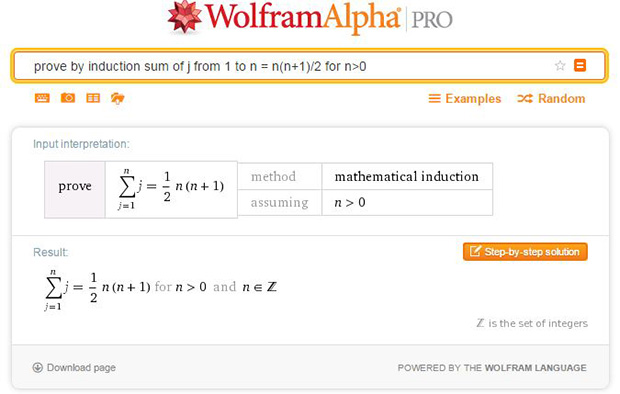#### Motivation

It is no secret that Wolfram|Alpha is a well-used tool for math students, especially those taking first-year college and university math courses. In fact, one of the most common responses I receive from students when I explain my role with Wolfram|Alpha is, “OMG, that site saved me during calculus.” This is usually a reference to the differential equation solver, or derivative and integral features of Wolfram|Alpha.

A majority of math topics that are taught at the high-school and first-year level are computation based, similar to the features listed above. This means questions on tests and exams are often in the following form:

• Solve for x
• Find the derivative of f(x)
• Determine the roots of the following equation

Computation questions typically involve performing a series of calculations (i.e. steps) in order to obtain a final result. Topics that can be solved computationally can often be done so using repetitive approaches. Once a student has mastered the limited steps and rules associated with one of these topics, they should be able to handle just about any problem that is thrown at them on an assignment or an exam related to that topic. This is because computation-based questions are generally consistent in the approaches used to solve them. Similar to learning how to calculate derivatives of functions, once a student has gained experience with all the necessary “derivative rules,” answering these questions is a matter of repeatedly applying those rules.

The problem, though, is what about topics that are not computation based? More specifically, how do students study and practice questions for math topics that don’t have a limited set of rules or approaches? I encountered this problem as a student in a first-year discrete math course. The topic being taught was proofs by principle of mathematical induction, which I will give a brief background on. This topic was completely different than anything I had previously been taught in math, for a couple reasons:

• Proofs are not computation questions. The same set of rules can’t be applied to every question.
• Proofs don’t have a single final answer.

It’s weird to think of a math problem that doesn’t have a nice, simple, clean answer at the bottom of the page. If a student was solving an equation on a test, they might use five lines to show their steps, and then at the very end they would likely have the final answer written in the form “x = 21.” Mathematical proofs, however, don’t work that way. In fact, the “answer” actually is the steps. The goal of a proof is to logically show why something is true.

Consider if you were playing the game Clue (a.k.a. Cluedo), a murder mystery board game. The goal of the game is to determine who the murderer is, the weapon used, and the location of the crime. Someone would therefore win by stating something along the lines of, “Colonel Mustard with the revolver in the dining room.” Now imagine instead of trying to figure out this information, you were told who the murderer was, the weapon used, and the location of the crime at the very beginning of the game. The goal now is then not to determine the conclusion (since you were already told it) but instead figure out why this is true. Based on your own cards and conclusions made from information being revealed throughout the game, you should eventually be able to prove using logic why the information you were told at the beginning of the game must be true. Therefore, you might win the game by stating something along the lines of, “Since I have all of the weapon cards besides the revolver, and Joe has all the location cards except the dining room, and I know neither of you have Colonel Mustard based on your cards played, I have proved the proposed murderer to be true.”

I am not at all suggesting that the above example would be a fun alteration to Clue; in fact, it would likely kill the fun of the game (bad joke). However, it demonstrates the type of question/answer format that proofs represent. Below is a sample induction proof question a first-year student might see on an exam:

Prove using mathematical induction that 8^n – 3^n is divisible by 5, for n > 0.

The assertion made, that 8^n – 3^n is divisible by 5 when n is greater than 0, is completely true (assuming n is an integer). However, the question is asking the student to logically show why the statement is correct. Just like in that alternate Clue example, you were told the information at the beginning of the game and then asked to prove why it’s true. Writing these proofs is not trivial for most students, and is often viewed as one of the more difficult math concepts to master.

Due to the complexity of these questions, students often rely on online resources for further help (e.g. Wolfram|Alpha). If students had a differential equation they were solving and wanted to verify their answer, they can easily input it into Wolfram|Alpha and be given the final answer as well as the step-by-step solution. This approach can also be taken for derivatives, integrals, simplifying expressions, etc.

We’ve established that proofs are not considered to be computation questions. As a result, to my understanding, no calculator or online tool has been able to generate solutions for proof questions… until now.

I vividly remember in my first year wanting to verify my solutions for proofs on an assignment. I had written out what I thought were the correct solutions to the proof questions. But how could I check if my answers were right? Surprisingly, there were only two ways to verify the correctness of my proofs:

• Find the exact same proof as an example online (which was very rare)
• Compare my solutions with someone else in the class, and assume if we both had the same solution that we must both be right (bad assumption)

So why is it so easy to find a “derivative calculator” online, but not a “proof calculator”?

The answer is mainly due to the fact that proofs have generally not been considered computable. Since the same set of rules can’t be applied to cover 100% of proofs, a computer has difficulty creating the logical steps of which the proof is composed. My solution to this problem was to build a library of pattern-matched proofs (as explained further below). This approach demonstrated the existence of computation playing a role in constructing proofs, and led to the creation of a working “proof generator.”

#### Prototype

Over a summer I began building a prototype application, which was able to perform a limited number of proofs using the principle of mathematical induction (PMI). PMI is a common tactic for constructing proofs. The logic for proving a query true via PMI is often compared to proving that a line of dominoes will all fall down in succession. First, you want to prove that the first domino will fall over if you push it. Second, you want to prove that if any given domino falls over, the one following it will also fall over. By proving these two facts, you are able to show that a line of dominoes will fall over if the first one is pushed.

This domino example well describes the logic behind induction proofs, which are composed of:

• Base case (first domino): Prove the statement is true for the base case (the smallest n value), i.e. if you were proving something was true for n ≥ 1, the base case is showing the statement is true for n = 1.
• Inductive step (consecutive dominoes): This is the more challenging step. In the inductive step, you assume the statement is true for some value (i.e. k), and then try to show this is also true for k + 1.

If both of these steps are done correctly, then the proof is complete.

Before development, I had discussed building this application with a professor at my undergraduate university (Algoma University). He compared this challenging idea to building a recipe book that contains every recipe in the world. You can keep adding as many recipes as you can get your hands on, but it is essentially impossible to include every recipe ever made. Similarly, I can keep adding more coverage for proofs to be generated, but I will never be able to include every proof that a user will ask for.

While this was a rather notable limitation, I thought about it in a slightly different sense. Instead I considered that if someone new to cooking wanted a cookbook, it might be a fairly large cookbook. There’s a wide range of recipes that they would want to try and make. At the same time, they would not likely be relying on a recipe for Gordon Ramsay’s Beef Wellington to be included in the book. The level of difficulty for preparing that dish is likely too high for someone who is brand-new to cooking. As for someone who is new to learning proofs (first-year university level), they likely won’t need access to a resource that generates very advanced proofs. As long as their resource can just cover queries used at the first-year level, then in their eyes the resource has terrific coverage. This was an important distinction made in the planning stage.

During the development of the prototype, I quickly realized there was a rather large challenge right out of the gate. Many of the proofs I wanted the application to cover involved simplifying expressions, which can be a very complex program on its own to write. It didn’t make sense to reinvent the wheel, and this was when I first discovered Wolfram|Alpha’s APIs. Using its APIs, I could simply make calls directly to Wolfram|Alpha (i.e. simplify an expression), and return the result back to my application. I had a few specific questions about the usage of the APIs, and so I decided to just contact Wolfram|Alpha directly with my questions.

The helpful person at the other end answered my questions quickly, and then the conversation took a direction I didn’t expect. After I explained the little side project I was building, I was asked to demonstrate the application to a few different members at Wolfram|Alpha (after signing a nondisclosure agreement, of course). At this point the application was fairly limited in terms of the number of proofs it could handle, but it performed well as a prototype or proof of concept. The demonstration went well, but I was once again surprised at the direction the meeting took. It was just a couple weeks after the demonstration that I was offered—and then accepted—a position with Wolfram|Alpha as a Math Content Developer.

#### How the app works

I moved to the Wolfram|Alpha HQ in Champaign, Illinois, the following summer. There, I was able to work on several projects related to new math features being added to the site. However, my main project was to reimplement my induction proof program to become part of Wolfram|Alpha.

The goal of the project was that a student would be able to input any induction proof question they had in a first-year course. For that to become a reality, I scoured the internet and several textbooks to search for all of the induction proof problems I could find… there were a lot.

To be clear, this project was not a database of all induction questions I could find. For the program to work consistently for all proofs (even ones it hadn’t seen before), I needed to first generalize the types of induction proofs that students are being taught. The decision was made early on that the program would handle three main types of queries for proofs:

• Summation/product equalities, e.g. prove 1 + 2 + 3 +… + n = n(n + 1)/2, for n > 0
• Expression divisibility, e.g. prove 8^n – 3^n is divisible by 5, for n > 0
• Expression inequalities, e.g. prove 2^n > n^3, for n > 9

Using these three main types of proof queries, I then further broke down each of these query types into subsets of more specific types of proofs. Below is an example of what I mean by that.

Let’s say we want to write a proof for the following:

Prove 8^n – 3^n is divisible by 5, for n > 0

Instead of “hard-coding” the full solution of this proof into the application, I want to generalize it as much as I can so that it can possibly cover even more proofs. Therefore, I don’t add very specific proofs to the application, but instead add pattern-matched proofs.

In the expression 8^n – 3^n, I really don’t care all that much about the 8 and the 3. The reason I don’t care is because even if those numbers change, it’s not going to change the structure of the proof being generated. That means if a user instead asked to prove that 10^n – 3^n is divisible by 7, the exact same steps would be followed for the 8^n – 3^n example, just with different values. In order to take advantage of this, I would therefore write a proof solution for this type of query:

Prove a^nb^n is divisible by d, for n > x

By pattern matching the user’s input, if it is detected that their query is in this form (i.e. follows this pattern), then their proof will be generated according to this structure. The variables (a, b, n, d, x) are then extracted in order to provide the values for the generated proof.

This concept is then applied to many different structures for proof queries. The pattern-matching approach seemed efficient in quickly covering most of the example proof questions I came across online and in textbooks. This approach is what allows the application to generate proofs for queries it hasn’t seen before, so long as it matches the pattern of one of the structures the app is aware of (of which there are many).

However, specific pattern matching is really only used for the expression divisibility and expression inequalities query types. For the summation/product equalities, the application is theoretically able to handle 100% of these queries. There is a specific mathematical approach for summation/product equalities that can be followed, regardless of the right-hand expression of the equality or the contents of the summation or product. This approach works both for proofs and disproofs of summation equalities. This is a nice feature, since it ensures complete coverage of induction proofs for this query type, so long as Wolfram|Alpha doesn’t time out due to large input.

For expression divisibility, most generated proofs are based solely off pattern matching the input. There is, however, a somewhat general-case algorithm used as a last-ditch effort, in case the given expression was not successfully pattern matched. The algorithm performs a certain manipulation on the expression in an attempt to put the expression into a specific form. This form is simply the addition of two terms, where one term is the inductive hypothesis and the other term contains a coefficient that has the given divisor as a factor. If this manipulation is successful, then a proof can be generated. Otherwise, it will fail.

As for expression inequalities, all the generated proofs are pattern matched since I am unaware of any general-case algorithm to apply toward expression inequality proofs. It makes more sense to pattern match the difference between the two expressions. Not only is this beneficial for inequality manipulation but this is also a crucial step in case any terms need to be eliminated. For example, here is a possible query:

Prove 2n + 7 + sin(n) < (n +7)^2 + sin(n), for n > 0

The proof generated here would ideally be pattern matched for the following:

Prove a n + c < (n + b)^d, for n > g

The problem is that the pattern-matched expression doesn’t include a sin(n) on each side of the inequality. However, if the difference between each side of the expression (e.g. left-hand side – right-hand side) is taken, the new query would just be 2n + 7 – (n+7)^2, which would be pattern matched against a n + c – (n+b)^d, which matches correctly.

It is also important to note that some of these variables can sometimes be optional (i.e. equal to 0 or 1), such as the constants, coefficients, and exponents.

Once testing for the application began, I noticed there were two types of queries that were consistently generating incorrect proofs. While the goal is to include coverage for as many proofs as possible, it is much, much, much worse to generate a proof that is mathematically incorrect as opposed to not generating a proof at all. As soon as the application starts producing invalid content, the quality and reliability of the application goes down the drain.

The first type of query that generated invalid proofs can be demonstrated with the following example:

Prove 4n + 7 > 2^n, for n ≥ 0

In this example, the base case (n = 0) correctly passes (since 7 > 1). However, the inductive step should fail because the actual claim being made is false (i.e. when n = 5, since 27 ≱ 32). But for whatever reason, the app attempted to generate an inductive step to make the proof valid. This resulted in faulty mathematics, and needed to be eliminated. Proofs using induction are terrific for verifying claims, but they are not so great for disproving claims. The decision was made then to not generate proofs (or “disproofs”) for expression inequality queries where the base case is valid but the given query is false.

The other type of query that generated invalid proofs can be explained using this example:

Prove 4n < 2^n, for n ≥ 0

This is actually a fairly tricky example. The graph below illustrates the comparison of these expressions: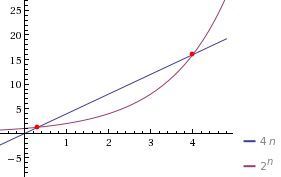The base case of this example is n = 0, which results in 4(0) < 2(0), which simplifies to 0 < 1, which is true. Since the base case passes, the inductive step can proceed. Like the previous example, this query also generated an invalid inductive step. The problem is that many of the manipulations that are done in the inductive step may be assuming a certain range of k. For example, in the above proof, one of the steps relies on the fact 3 < 2^k, for k ≥ 2. However, the range of k values between the base case and k ≥ 2, where n = k, has not been proven to satisfy the given claim of
“Prove 4n < 2^n”. Therefore, the base case must now also include n = 1 and n = 2 in the base case coverage. Note that a “modified proof” would need to suggest that n > 4 since the case that n = 4 is not true for 4n < 2^n.

If a manipulation is made in the inductive step that requires n ≥ 2 but the base case is n = 0, then we haven’t proven the query to be true for n = 1 or n = 2. This is very similar to the false paradox “all horses are the same color.” The solution to this problem was not to get rid of the proof altogether. Instead, the base case step would test multiple values of n if any assumption was to be made in the inductive step. Therefore, the query 4n < 2^n would fail in the base case because it is not true for
n = 1.

The final challenge with this project involved the natural language processing (NLP). This allows a user to ask a question in plain English and have Wolfram|Alpha understand what they’re looking for. While I had no applicable experience with NLP before, the existing parsing/scanning framework on Wolfram|Alpha made it fairly easy to integrate the NLP component with the application. The main challenge was trying to determine all the possible ways a user can ask Wolfram|Alpha to prove a query using induction. This continues to be an ongoing development, as different phrasings for queries are still being added.

#### Using the app

Here’s a screen shot of the application on Wolfram|Alpha: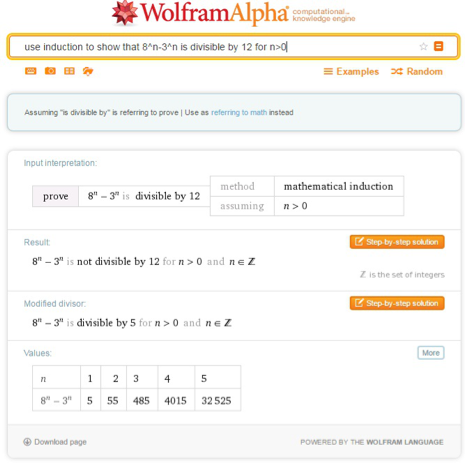By clicking on the “Step-by-step solution” button (a Wolfram|Alpha Pro feature), you will be able to see the generated proof. All users, however, will be able to see the above content. Additional information has been added to the application in order to provide important details to the user. A feature added to this result page is that a different divisor is suggested when the proof is invalid.

Below is a screen shot of the generated proof with the “modified divisor”: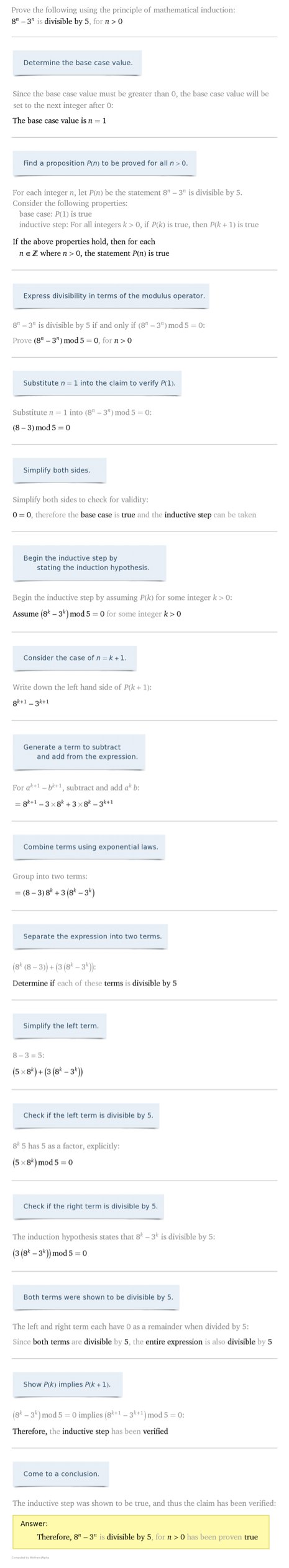For the multiple-base case example discussed, two proofs were generated for this result—one that shows the initial proof (invalid), and then a second proof that recommends a different base case in order to make the proof valid: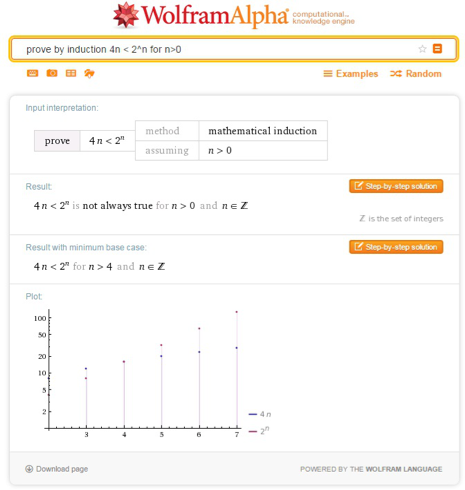One final, cool feature is that an invalid summation/product equality proof will be corrected with a new recommended expression: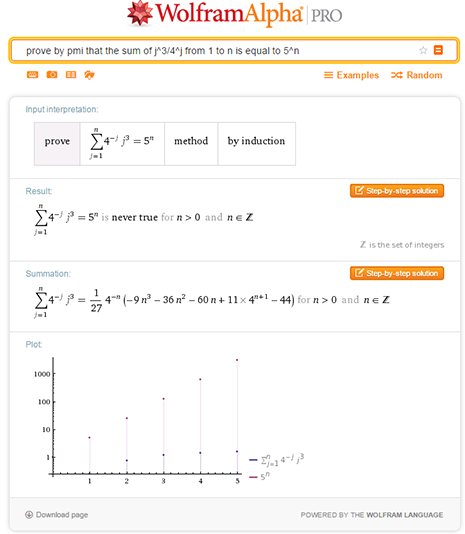#### Aftermath

The application went live on Wolfram|Alpha on October 15, 2015 (I’ll never forget it). This was approximately fifteen months after I began designing the prototype program. This date was also six days before I presented the application at the Wolfram Technology Conference on my twenty-first birthday. The success and feedback from this project has been more than I ever could have imagined.

By taking an idea and some initiative, I landed a position with the world’s largest computational knowledge engine (where I’ve been an employee for more than a year now). This initiative also played a key role in being accepted as a graduate student in the University of Waterloo’s symbolic computation department.

While the goal of the project was to generate induction proofs, this whole process was a terrific proof for the rewards that can result from imagination, originality, and hard work.